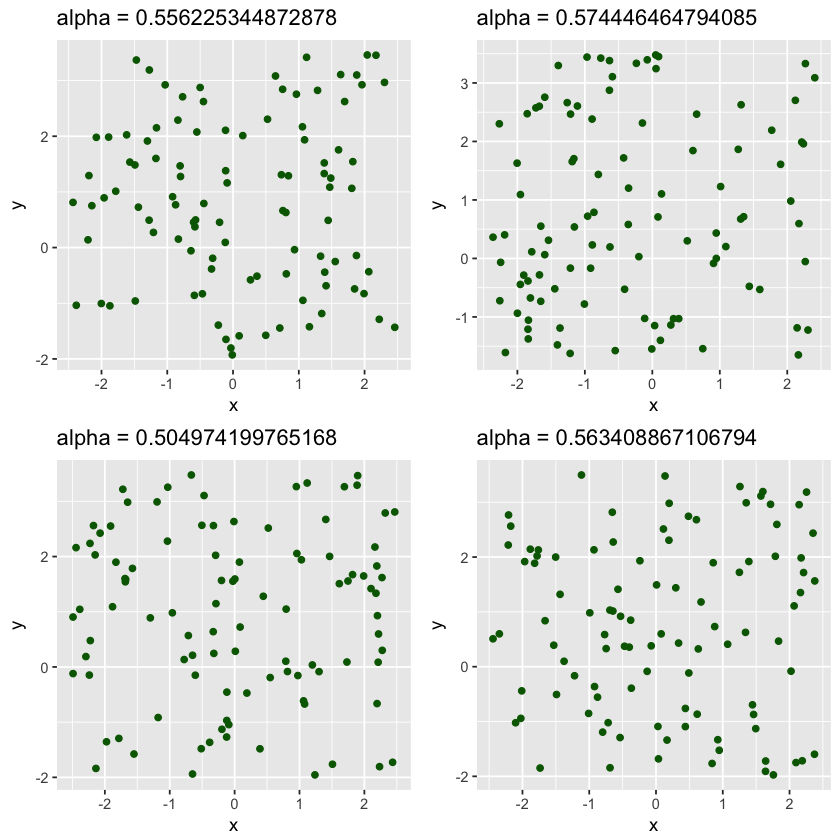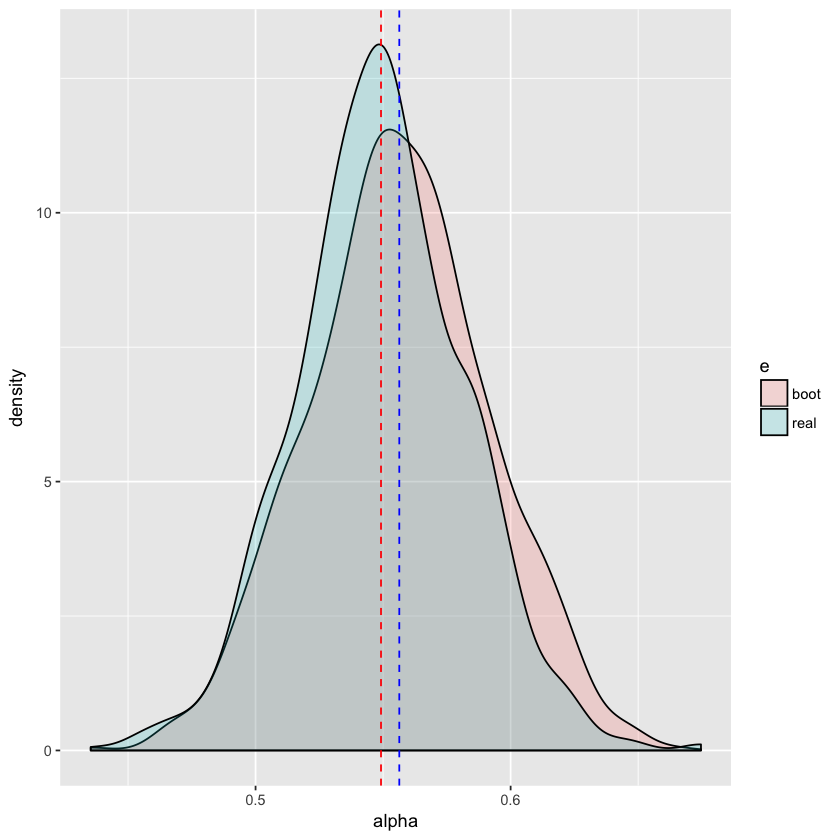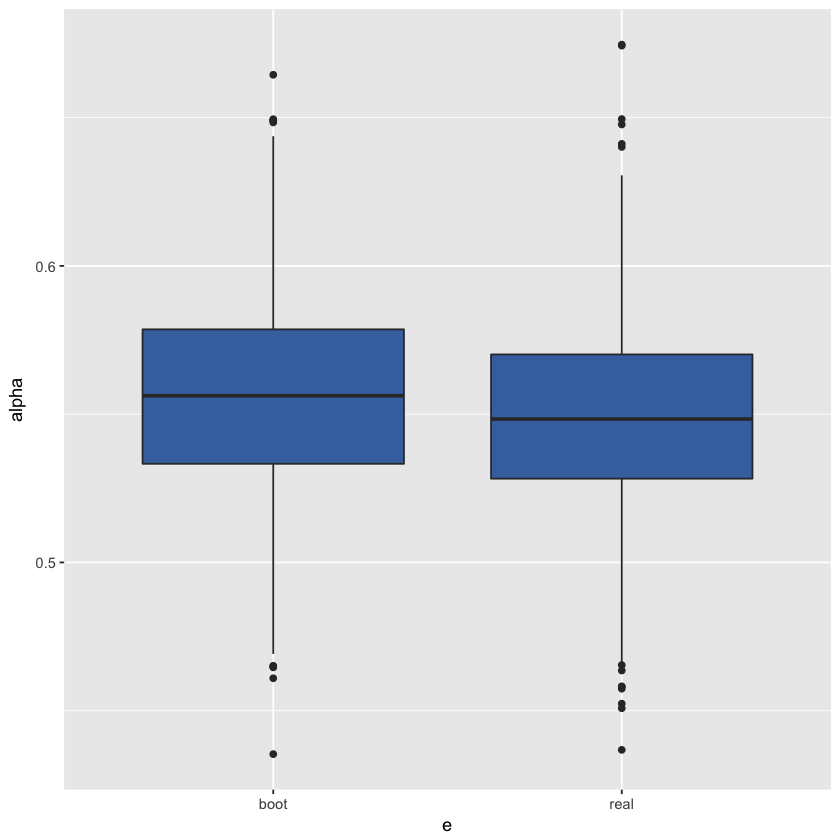# Bootstrap in R¶

### Elan Ding¶

Aug. 26, 2018 $\newcommand{\bs}{\boldsymbol}$ $\newcommand{\argmin}{\underset{\bs{#1}}{\text{arg min}}\,}$ $\newcommand{\argmax}{\underset{\bs{#1}}{\text{arg max}}\,}$ $\newcommand{\tr}{^{\top}}$ $\newcommand{\norm}{\left|\left|\,#1\,\right|\right|}$ $\newcommand{\given}{\,|\,}$

R is a great language for quick prototyping. For statistical analysis, it is the best option out there. In this post we will be using R to do some visualizations using ggplot2.

Supppose we wish to invest in two stocks $X$ and $Y$, with risk $\sigma_X$ and $\sigma_Y$ measured by their standard deviations. As an investor, we want to minimize the risk of investing in both stocks. Let $\alpha$ be a number in $[0, 1]$. We will invest a fraction $\alpha$ of our money in $X$ and the remaining $1-\alpha$ in $Y$. So we want to minimize

$$\text{Var}(\alpha X + (1-\alpha)Y).$$

This can be rewritten as

\begin{aligned} &\text{Var}(\alpha X) + \text{Var}((1-\alpha)Y) + 2\text{Cov}(\alpha X, (1-\alpha) Y) \\ =& \, \alpha^2 \sigma_X^2 + (1-\alpha)^2 \sigma_Y^2 + 2\alpha(1-\alpha)\sigma_{XY} \end{aligned}

where $\sigma_X$ and $\sigma_Y$ are the variances of $X$ and $Y$, and $\sigma_{XY}$ is the covariance of $X$ and $Y$.

This quantity can be easily minimized by setting the derivative to 0:

$$2\alpha \sigma_X^2 - 2(1-\alpha)\sigma_Y^2 + 2\sigma_{XY} - 4\alpha \sigma_{XY} = 0$$

Solving this we obtain

$$\alpha = \frac{\sigma_Y^2 - \sigma_{XY}}{\sigma_X^2 + \sigma_Y^2 - 2\sigma_{XY}} \tag{1}$$

In R this function can be implemented as follows.

In :
alpha=function(x,y){
vx=var(x)
vy=var(y)
cxy=cov(x,y)
(vy-cxy)/(vx+vy-2*cxy)
}


The stock $X$ will be a uniformly distributed in the interval $[-2.5, 2.5]$, so

\begin{aligned} E(X) & = \frac{2.5-2.5}{2} = 0 \\ \text{Var}(X) &= \frac{(2.5- (-2.5))^2}{12} \approx 2.08 \end{aligned}

On the other hand, the stock $Y$ will be uniformly distributed in $[-2, 3.5]$, so

\begin{aligned} E(Y) & = \frac{3.5-2}{2} = 0.75 \\ \text{Var}(Y) &= \frac{(3.5- (-2))^2}{12} \approx 2.52 \end{aligned}

The covariance here is 0 since $X$ and $Y$ are independent by construction. Hence the true value of $\alpha$ is

$$\alpha = \frac{\sigma_Y^2}{\sigma_X^2 + \sigma_Y^2} \approx 0.5475.$$

However, in the real world, the true risk of a stock is never known. Instead, we take historical data and use the sample standard deviation as an estimator. In R, we can simulate stocks $X$ and $Y$ as follows.

In :
set.seed(1)

# scatterplot
require(ggplot2)
require(grid)
require(gridExtra)

plots <- list()
for (i in 1:4) {
x <- runif(100, -2.5, 2.5)
y <- runif(100, -2, 3.5)
a <- alpha(x, y)
plots[[i]] <- ggplot(mapping=aes_(x, y)) +
geom_point(color='darkgreen') +
ggtitle(paste0("alpha = ", a)) +
labs(x = "x", y="y")
}

grid.arrange(grobs=plots, ncol = 2)So we got alpha values around 0.5. How can we find a confidence interval for $\alpha$? In this case, it is very easy to run many simulations and estimate the convidence interval using the sample variance.

In :
# define a row vector of 0's
a <- list()

# simulate 1000 stock data
set.seed(2)
for (i in 1:1000) {
x <- runif(100, -2.5, 2.5)
y <- runif(100, -2, 3.5)
a[[i]] <- alpha(x, y)
}

a <- as.numeric(a)
a.n <- length(a)
a.sd <- sd(a)
a.mean <- mean(a)

# Constructing 90% confidence interval using the t-distribution
a.se <- qt((0.90+1)/2, df = a.n - 1) * a.sd / sqrt(a.n)
result <- c("lower" = a.mean - a.se, "upper" = a.mean + a.se)
print(result)

    lower     upper
0.5474690 0.5508967


This means that based on the 100 simulated data, we are 90% confident that the true $\alpha$ is between 0.547 and 0.550. Indeed this is the case here. The sampling distribution is plotted below. The red vertical line is the true value of $\alpha$, and the blue lines are one standard deviation away from the mean.

In :
require(dplyr)

hist(a) %>% abline(v=mean(a), col="red")## The Bootstrap¶

However, in real life, we can't simulate stock data. We don't have a population to simulate from; instead, we only have a single sample. Bootstrap uses a clever idea of sampling not from the population, but directly from the data with replacement. Each time we create a bootstrapped sample, we get one estimate of $\alpha$, and after we repeat this process for many times, we get an estimated sampling distribution.

In :
# simulate X and Y the same as before
set.seed(1)
x <- runif(100, -2.5, 2.5)
y <- runif(100, -2, 3.5)

# creating a dataframe of the stock
portfolio <- data.frame(x=x, y=y)


Based on the portfolio created above, the point estimate for the $\alpha$ value can be found.

In :
alpha(portfolio$x, portfolio$y)

0.556225344872878

Now we are ready to use bootstrap to estimate the confidence interval of our estimate.

In :
# create a function that calculates the alpha value based on the index chosen
alpha.fn = function(data, index){
with(data[index,], alpha(x, y))
}

# bootstrap function that returns an array of alpha statistics
bootstrap = function(portfolio, iterations){
set.seed(1)
alpha <- list()
for (i in 1:iterations){
alpha[[i]] <- alpha.fn(portfolio, sample(1:100, 100, replace=TRUE))
}
return(as.numeric(alpha))
}

# create a bootstrap sample of size 1000
aa = bootstrap(portfolio, 1000)

# converting matrix to data frame to make ggplot work better
real <- data.frame(alpha = a)
boot <- data.frame(alpha = aa)

# adding an extra column that distinguishes them
real$e <- 'real' boot$e <- 'boot'

# combine into a single data frame
combo <- rbind(real, boot)


alphae
0.5649038real
0.5582860real
0.5618855real
0.5892127real
0.5452870real
0.5384885real
In :
# plotting a histogram
ggplot(combo, aes(alpha, fill=e)) + geom_density(alpha = 0.2) +
geom_vline(aes(xintercept = mean(a)), color="red", linetype="dashed") +
geom_vline(aes(xintercept = mean(aa)), color="blue", linetype="dashed")In :
# plotting a boxplot
ggplot(combo, aes(x=e, y=alpha)) + geom_boxplot(fill="#4271AE")We see that the bootstrap distribution is very close to the real sampling distribution.